# dendrogram

Dendrogram plot

## Syntax

``dendrogram(tree)``
``dendrogram(tree,Name,Value)``
``dendrogram(tree,P)``
``dendrogram(tree,P,Name,Value)``
``H = dendrogram(___)``
``````[H,T,outperm] = dendrogram(___)``````

## Description

example

````dendrogram(tree)` generates a dendrogram plot of the hierarchical binary cluster tree. A dendrogram consists of many U-shaped lines that connect data points in a hierarchical tree. The height of each U represents the distance between the two data points being connected.If there are 30 or fewer data points in the original data set, then each leaf in the dendrogram corresponds to one data point. If there are more than 30 data points, then `dendrogram` collapses lower branches so that there are 30 leaf nodes. As a result, some leaves in the plot correspond to more than one data point.```

example

````dendrogram(tree,Name,Value)` uses additional options specified by one or more name-value pair arguments.```

example

````dendrogram(tree,P)` generates a dendrogram plot with no more than `P` leaf nodes. If there are more than `P` data points in the original data set, then `dendrogram` collapses the lower branches of the tree. As a result, some leaves in the plot correspond to more than one data point.```
````dendrogram(tree,P,Name,Value)` uses additional options specified by one or more name-value pair arguments.```

example

````H = dendrogram(___)` generates a dendrogram plot and returns a vector of line handles. You can use any of the input arguments from the previous syntaxes.```

example

``````[H,T,outperm] = dendrogram(___)``` also returns a vector containing the leaf node number for each object in the original data set, `T`, and a vector giving the order of the node labels of the leaves as shown in the dendrogram, `outperm`. It is useful to return `T` when the number of leaf nodes, `P`, is less than the total number of data points, so that some leaf nodes in the display correspond to multiple data points.The order of the node labels given in `outperm` is from left to right for a horizontal dendrogram, and from bottom to top for a vertical dendrogram. ```

## Examples

collapse all

Generate sample data.

```rng('default') % For reproducibility X = rand(10,3);```

Create a hierarchical binary cluster tree using `linkage`. Then, plot the dendrogram using the default options.

```tree = linkage(X,'average'); figure() dendrogram(tree)```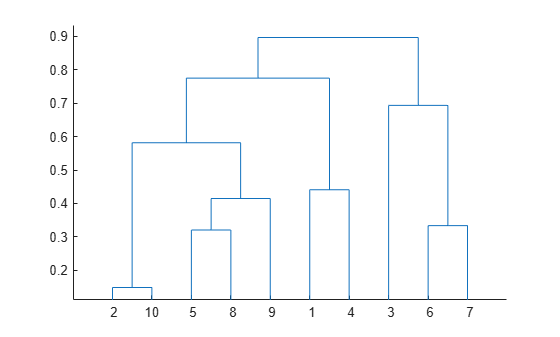Generate sample data.

```rng('default') % For reproducibility X = rand(10,3);```

Create a hierarchical binary cluster tree using `linkage`.

```tree = linkage(X,'average'); D = pdist(X); leafOrder = optimalleaforder(tree,D)```
```leafOrder = 1×10 3 7 6 1 4 9 5 8 10 2 ```

Plot the dendrogram using an optimal leaf order.

```figure() dendrogram(tree,'Reorder',leafOrder)```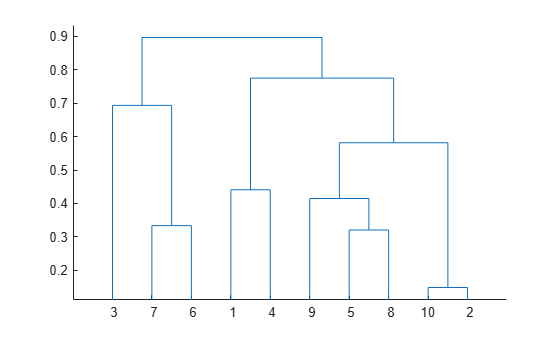The order of the leaf nodes in the dendrogram plot corresponds - from left to right - to the permutation in `leafOrder`.

Generate sample data.

```rng('default') % For reproducibility X = rand(100,2);```

There are 100 data points in the original data set, `X`.

Create a hierarchical binary cluster tree using `linkage`. Then, plot the dendrogram for the complete tree (100 leaf nodes) by setting the input argument `P` equal to `0`.

```tree = linkage(X,'average'); dendrogram(tree,0)```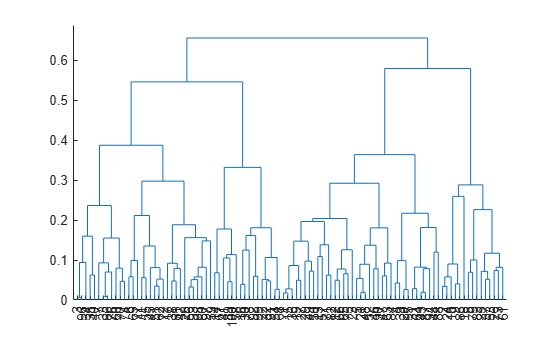Now, plot the dendrogram with only 25 leaf nodes. Return the mapping of the original data points to the leaf nodes shown in the plot.

```figure [~,T] = dendrogram(tree,25);```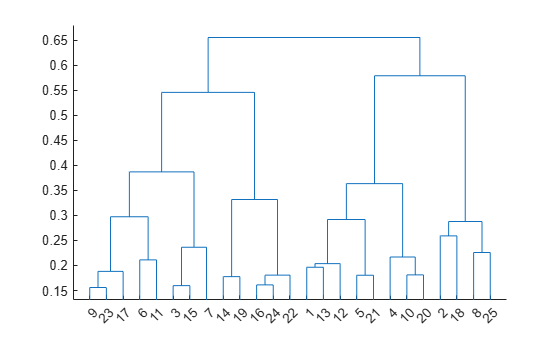List the original data points that are in leaf node 7 of the dendrogram plot.

`find(T==7)`
```ans = 7×1 7 33 60 70 74 76 86 ```

Generate sample data.

```rng('default') % For reproducibility X = rand(10,3);```

Create a hierarchical binary cluster tree using `linkage`. Then, plot the dendrogram with a vertical orientation, using the default color threshold. Return handles to the lines so you can change the dendrogram line widths.

```tree = linkage(X,'average'); H = dendrogram(tree,'Orientation','left','ColorThreshold','default'); set(H,'LineWidth',2)```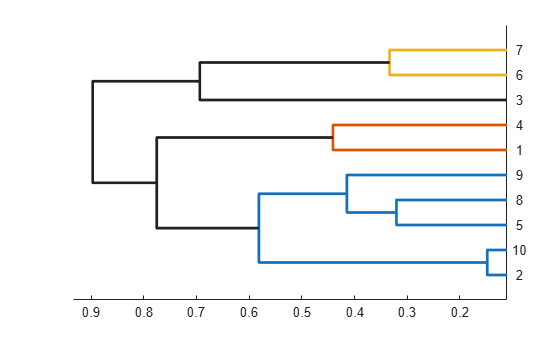## Input Arguments

collapse all

Hierarchical binary cluster tree, specified as an (M – 1)-by-3 matrix that you generate using `linkage`, where M is the number of data points in the original data set.

Maximum number of leaf nodes to include in the dendrogram plot, specified as a positive integer value.

• If there are `P` or fewer data points in the original data set, then each leaf in the dendrogram corresponds to one data point.

• If there are more than `P` data points, then `dendrogram` collapses lower branches so that there are `P` leaf nodes. As a result, some leaves in the plot correspond to more than one data point.

If you do not specify `P`, then `dendrogram` uses 30 as the maximum number of leaf nodes. To display the complete tree, set `P` equal to `0`.

Data Types: `single` | `double`

### Name-Value Pair Arguments

Specify optional comma-separated pairs of `Name,Value` arguments. `Name` is the argument name and `Value` is the corresponding value. `Name` must appear inside quotes. You can specify several name and value pair arguments in any order as `Name1,Value1,...,NameN,ValueN`.

Example: `'Orientation','left','Reorder',myOrder` specifies a vertical dendrogram with leaves in the order specified by `myOrder`.

Order of leaf nodes in the dendrogram plot, specified as the comma-separated pair consisting of `'Reorder'` and a vector giving the order of nodes in the complete tree. The order vector must be a permutation of the vector `1:M`, where M is the number of data points in the original data set. Specify the order from left to right for horizontal dendrograms, and from bottom to top for vertical dendrograms.

If M is greater than the number of leaf nodes in the dendrogram plot, `P` (by default, `P` is 30), then you can only specify a permutation vector that does not separate the groups of leaves that correspond to collapsed nodes.

Data Types: `single` | `double`

Indicator for whether to check for crossing branches in the dendrogram plot, specified as the comma-separated pair consisting of `'CheckCrossing'` and either `true` or `false`. This option is only useful when you specify a value for `Reorder`.

When `CheckCrossing` has the value `true`, `dendrogram` issues a warning if the order of the leaf nodes causes crossing branches in the plot. If the dendrogram plot does not show a complete tree (because the number of data points in the original data set is greater than `P`), `dendrogram` only issues a warning when the order of the leaf nodes causes branch to cross in the dendrogram as shown in the plot. That is, there is no warning if the order causes crossing branches in the complete tree but not in the dendrogram as shown in the plot.

Data Types: `logical`

Threshold for unique colors in the dendrogram plot, specified as the comma-separated pair consisting of `'ColorThreshold'` and either `'default'` or a scalar value in the range `(0,max(tree(:,3)))`. If `ColorThreshold` has the value T, then `dendrogram` assigns a unique color to each group of nodes in the dendrogram whose linkage is less than T.

• If `ColorThreshold` has the value `'default'`, then the threshold, T, is 70% of the maximum linkage, `0.7*max(tree(:,3))`.

• If you do not specify a value for `ColorThreshold`, or if you specify a threshold outside the range `(0,max(tree(:,3)))`, then `dendrogram` uses only one color for the dendrogram plot.

Orientation of the dendrogram in the figure window, specified as the comma-separated pair consisting of `'Orientation'` and one of these values:

 `'top'` Top to bottom `'bottom'` Bottom to top `'left'` Left to right `'right'` Right to left

Label for each data point in the original data set, specified as the comma-separated pair consisting of `'Labels'` and a character array, string array or cell array of character vectors. `dendrogram` labels any leaves in the dendrogram plot containing a single data point with that data point’s label.

## Output Arguments

collapse all

Handles to lines in the dendrogram plot, returned as a vector.

Leaf node numbers for each data point in the original data set, returned as a column vector of length M, where M is the number of data points in the original data set.

When there are fewer than `P` data points in the original data (`P` is 30, by default), all data points are displayed in the dendrogram, with each node containing a single data point. In this case, `T` is the identity map, `T = (1:M)'`.

`T` is useful when `P` is less than the total number of data points. That is, when some leaf nodes in the dendrogram display correspond to multiple data points. For example, to find out which data points are contained in leaf node `k` of the dendrogram plot, use `find(T==k)`.

Permutation of the node labels of the leaves of the dendrogram as shown in the plot, returned as a row vector. `outperm` gives the order from left to right for a horizontal dendrogram, and from bottom to top for a vertical dendrogram. If there are `P` leaves in the dendrogram plot, `outperm` is a permutation of the vector `1:P`.